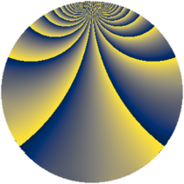# Properties

 Label 1386.2.clLevel $1386$ Weight $2$ Character orbit 1386.cl Rep. character $\chi_{1386}(481,\cdot)$ Character field $\Q(\zeta_{30})$ Dimension $768$ Sturm bound $576$

# Related objects

## Defining parameters

 Level: $$N$$ $$=$$ $$1386 = 2 \cdot 3^{2} \cdot 7 \cdot 11$$ Weight: $$k$$ $$=$$ $$2$$ Character orbit: $$[\chi]$$ $$=$$ 1386.cl (of order $$30$$ and degree $$8$$) Character conductor: $$\operatorname{cond}(\chi)$$ $$=$$ $$693$$ Character field: $$\Q(\zeta_{30})$$ Sturm bound: $$576$$

## Dimensions

The following table gives the dimensions of various subspaces of $$M_{2}(1386, [\chi])$$.

Total New Old
Modular forms 2368 768 1600
Cusp forms 2240 768 1472
Eisenstein series 128 0 128

## Trace form

 $$768q + 192q^{4} + 12q^{9} + O(q^{10})$$ $$768q + 192q^{4} + 12q^{9} + 4q^{11} + 12q^{15} - 192q^{16} + 12q^{23} - 96q^{25} + 36q^{26} + 54q^{27} - 50q^{35} + 8q^{36} + 20q^{39} - 180q^{41} - 40q^{42} - 4q^{44} - 48q^{45} + 40q^{51} + 52q^{53} + 40q^{57} - 18q^{58} + 8q^{60} - 110q^{63} + 192q^{64} - 24q^{66} + 12q^{70} + 32q^{71} - 40q^{72} - 84q^{75} + 30q^{77} + 48q^{78} + 52q^{81} - 20q^{84} + 8q^{86} + 60q^{89} + 18q^{92} + 66q^{93} - 160q^{95} + 114q^{99} + O(q^{100})$$

## Decomposition of $$S_{2}^{\mathrm{new}}(1386, [\chi])$$ into newform subspaces

The newforms in this space have not yet been added to the LMFDB.

## Decomposition of $$S_{2}^{\mathrm{old}}(1386, [\chi])$$ into lower level spaces

$$S_{2}^{\mathrm{old}}(1386, [\chi]) \cong$$ $$S_{2}^{\mathrm{new}}(693, [\chi])$$$$^{\oplus 2}$$